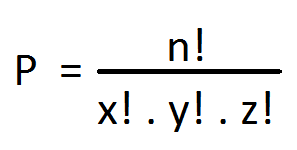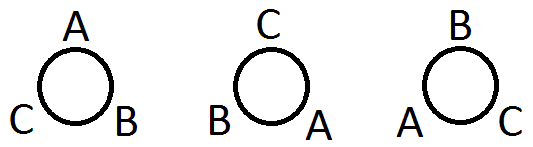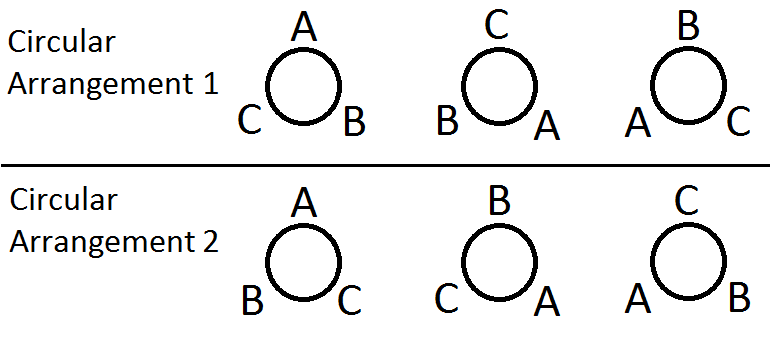# Circular Permutation

Aptitude

Before diving into circular permutation let us discuss Permutation of n things not all different taken all together. If we have n things of which x number of things are of same kind, y number of things are of same type and similarly z number of things are of the same type.

In this case the required number of permutations is written as,

P = n! / (x! y! z!)Let’s take a question.

Q. In how many ways can the letters of the word “GOOGLE” be arranged?

A. In this case we have 6 letters of which 2 are O and 2 are G.

So total number of permutations in this case = 6! / (2! X 2!) = 180

Therefore, we can have 180 different arrangements.

Let’s take another question.

Q. There are 3 copies of Harry Potter and the Philosopher's Stone, 4 copies of The Lost Symbol, 5 copies of The Secret of the Unicorn. In how many ways can you arrange these books on a shelf?

A. Total number of books = 3 + 4 + 5 = 12

Of which 3 are Harry Potter, 4 are The Lost Symbols by Dan Brown and 5 are The Secrete of The Unicorn by Herge.

So the required number of permutation = 12! / (3! X 4! X 5!) = 27720 ways.

## Permutation where repetition is allowed

This is a very interesting part of permutation. Say for instance, you have the digits 0, 1, 2, 3, 4, 5, 6, 7, 8, and 9 and you are asked to find the total numbers of 6 digits passwords that can be formed using those 10 digits and repetition is allowed.

So we have to find the number of permutations of 10 digits taken 6 at a time with repetition allowed.

This means we have 6 places and 10 digits to fill those 6 places. Let’s mark these places as A, B, C, D, E and F

[ A | B | C | D | E | F ]

We can fill the first place i.e., A with any one of the 10 digits.

Similarly, we can fill the second place i.e., B with any one of the 10 digits as repetition is allowed so we can reuse the digit used in A.

Proceeding in a similar way C, D, E and F can be filled with any one of the 10 digits respectively.

So we have 6 places and each of the places can be filled with any one of the 10 digits.

Therefore, the number of permutations in this case = 10x10x10x10x10x10 = 1000000

## Circular Permutation

Permutation in a circle is called circular permutation.

If we consider a round table and 3 persons then the number of different sitting arrangement that we can have around the round table is an example of circular permutation.

Circular permutation is a very interesting case. Let’s try to solve the above problem. If we have 3 persons and if we want to arrange them in a linear fashion then the total number of permutation of 3 persons taken all at a time is 3P3 = 3! = 6. Now for the sake of our convenience let us represent them as A, B and C. So we will have the following linear sitting arrangements

ABC

ACB

BAC

BCA

CAB

CBA

Now the circular part!

If we arrange these 3 persons around a round table as show in the picture below, we notice that all the different arrangements are not actually different, rather they all are same. How? Well… If you move clockwise, start with A, round the table in the picture shown below you will always get A-B-C. See for yourself.So it turns out that 3 linear permutations is actually 1 circular permutation.

Hence in general if we have n elements then total linear permutation of n elements taken all at a time is n! And we observe that n linear permutations correspond to 1 circular permutation.

So for n elements, circular permutation = n! / n = (n-1)!

Now if we solve the above problem, we get total number of circular permutation of 3 persons taken all at a time = (3-1)! = 2.So, in the above picture 3 linear arrangements makes 1 circular arrangement.

Linear arrangements ABC, CAB, BCA = Circular arrangement 1

Linear arrangements ACB, BAC, CBA = Circular arrangement 2

The point is in circular permutation one element is fixed and the remaining elements are arranged relative to it.

Now let me tell you something that is really interesting and if you have paid a closer attention to the above picture then you can guess what I want to tell.

Got the clue?

Well… If you consider JUST, and I want to emphasis this, if you JUST want to find the total number of circular permutation without taking into consideration the clockwise and counter clockwise issue then for 3 persons you get (3-1)! = 2 possible circular arrangements as show in the above picture. But if you consider the direction, the clockwise and counter clockwise order, then the scenario change. So if the clock clockwise and counter clockwise orders are not distinguishable then the total number of circular permutation of n elements taken all together = (n-1)! / 2.

Check it out. If you look at the above picture you will definitely notice that in the circular arrangement 1 if you move clockwise you get the result A-B-C. And in the circular arrangement 2 if you move counter clockwise you get the same result A-B-C.

So the important point about circular arrangement is as follows:

1. If the clockwise and counter clockwise orders CAN be distinguished then total number of circular permutation of n elements taken all together = (n-1)!
2. If the clockwise and counter clockwise orders CANNOT be distinguished then total number of circular permutation of n elements taken all together = (n-1)! / 2

Example of indistinguishable case is, if you consider 5 diamonds and you want to make a necklace. In this case 5 diamonds can be arranged in a circle in (5-1)! = 24 ways. But in case of forming a necklace the clockwise and counter clockwise arrangements cannot be distinguished. So the total circular permutation in this case = (5-1)! / 2 = 4! / 2 = 12 ways.